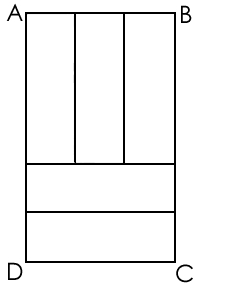## GMAT - Data Sufficiency - Test 4

1. #### 2x + y + 4z = 23

2. 3x + 4y + z = 22
A. statement 1 alone is sufficient, but statement 2 alone is not sufficient to answer the question
B. statement 2 alone is sufficient, but statement 1 alone is not sufficient to answer the question
C. both statements taken together are sufficient to answer the question, but neither statement alone is sufficient
D. each statement alone is sufficient
E. statements 1 and 2 together are not sufficient, and additional data is needed to answer the question

1. #### p ? q > 0

2. p > q
A. statement 1 alone is sufficient, but statement 2 alone is not sufficient to answer the question
B. statement 2 alone is sufficient, but statement 1 alone is not sufficient to answer the question
C. both statements taken together are sufficient to answer the question, but neither statement alone is sufficient
D. each statement alone is sufficient
E. statements 1 and 2 together are not sufficient, and additional data is needed to answer the question

1. #### x² + 2x = 0

2. x = -2

A. statement 1 alone is sufficient, but statement 2 alone is not sufficient to answer the question
B. statement 2 alone is sufficient, but statement 1 alone is not sufficient to answer the question
C. both statements taken together are sufficient to answer the question, but neither statement alone is sufficient
D. each statement alone is sufficient
E. statements 1 and 2 together are not sufficient, and additional data is needed to answer the question1. #### The perimeter of ABCD is 32.

2. All the small rectangles have the same dimensions.
A. statement 1 alone is sufficient, but statement 2 alone is not sufficient to answer the question
B. statement 2 alone is sufficient, but statement 1 alone is not sufficient to answer the question
C. both statements taken together are sufficient to answer the question, but neither statement alone is sufficient
D. each statement alone is sufficient
E. statements 1 and 2 together are not sufficient, and additional data is needed to answer the question

1. #### The team has three members.

2. Tom and Dick worked together for the whole of the previous day.
A. statement 1 alone is sufficient, but statement 2 alone is not sufficient to answer the question
B. statement 2 alone is sufficient, but statement 1 alone is not sufficient to answer the question
C. both statements taken together are sufficient to answer the question, but neither statement alone is sufficient
D. each statement alone is sufficient
E. statements 1 and 2 together are not sufficient, and additional data is needed to answer the question

1. #### y = x + 5

2. x = 6
A. statement 1 alone is sufficient, but statement 2 alone is not sufficient to answer the question
B. statement 2 alone is sufficient, but statement 1 alone is not sufficient to answer the question
C. both statements taken together are sufficient to answer the question, but neither statement alone is sufficient
D. each statement alone is sufficient
E. statements 1 and 2 together are not sufficient, and additional data is needed to answer the question

1. #### He gave his children \$2,000 each.

2. The wife received \$1,000 less than her husband and three thousand more than each of the children.
A. statement 1 alone is sufficient, but statement 2 alone is not sufficient to answer the question
B. statement 2 alone is sufficient, but statement 1 alone is not sufficient to answer the question
C. both statements taken together are sufficient to answer the question, but neither statement alone is sufficient
D. each statement alone is sufficient
E. statements 1 and 2 together are not sufficient, and additional data is needed to answer the question

1. #### x is a positive integer greater than 1.

2. |x|> 0
A. statement 1 alone is sufficient, but statement 2 alone is not sufficient to answer the question
B. statement 2 alone is sufficient, but statement 1 alone is not sufficient to answer the question
C. both statements taken together are sufficient to answer the question, but neither statement alone is sufficient
D. each statement alone is sufficient
E. statements 1 and 2 together are not sufficient, and additional data is needed to answer the question

1. #### x < 5/4

2. d < 5
A. statement 1 alone is sufficient, but statement 2 alone is not sufficient to answer the question
B. statement 2 alone is sufficient, but statement 1 alone is not sufficient to answer the question
C. both statements taken together are sufficient to answer the question, but neither statement alone is sufficient
D. each statement alone is sufficient
E. statements 1 and 2 together are not sufficient, and additional data is needed to answer the question

1. #### No team scored fewer points than C, which scored 11 points.

2. Team A won two events and came second in the third event.
A. statement 1 alone is sufficient, but statement 2 alone is not sufficient to answer the question
B. statement 2 alone is sufficient, but statement 1 alone is not sufficient to answer the question
C. both statements taken together are sufficient to answer the question, but neither statement alone is sufficient
D. each statement alone is sufficient
E. statements 1 and 2 together are not sufficient, and additional data is needed to answer the question